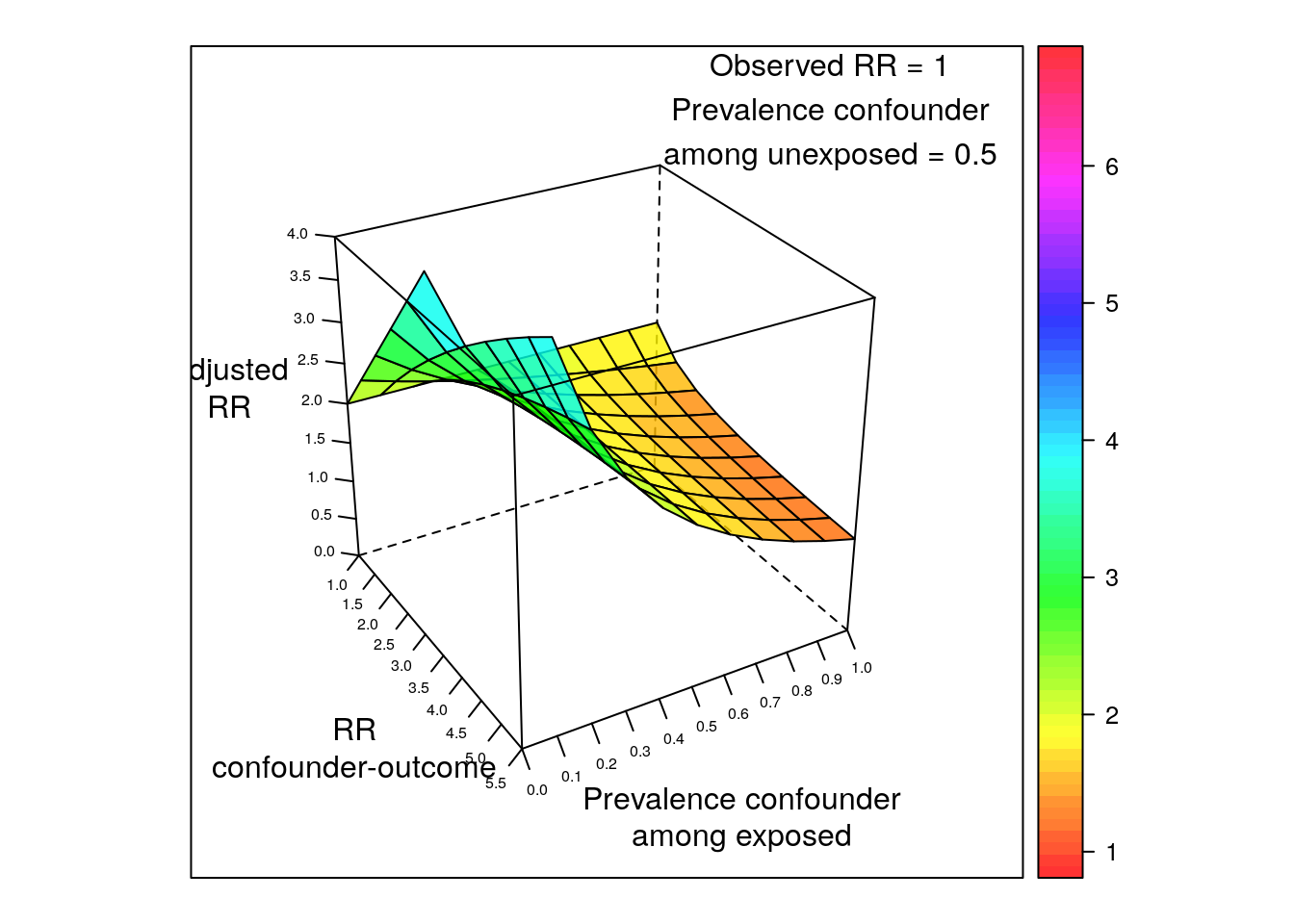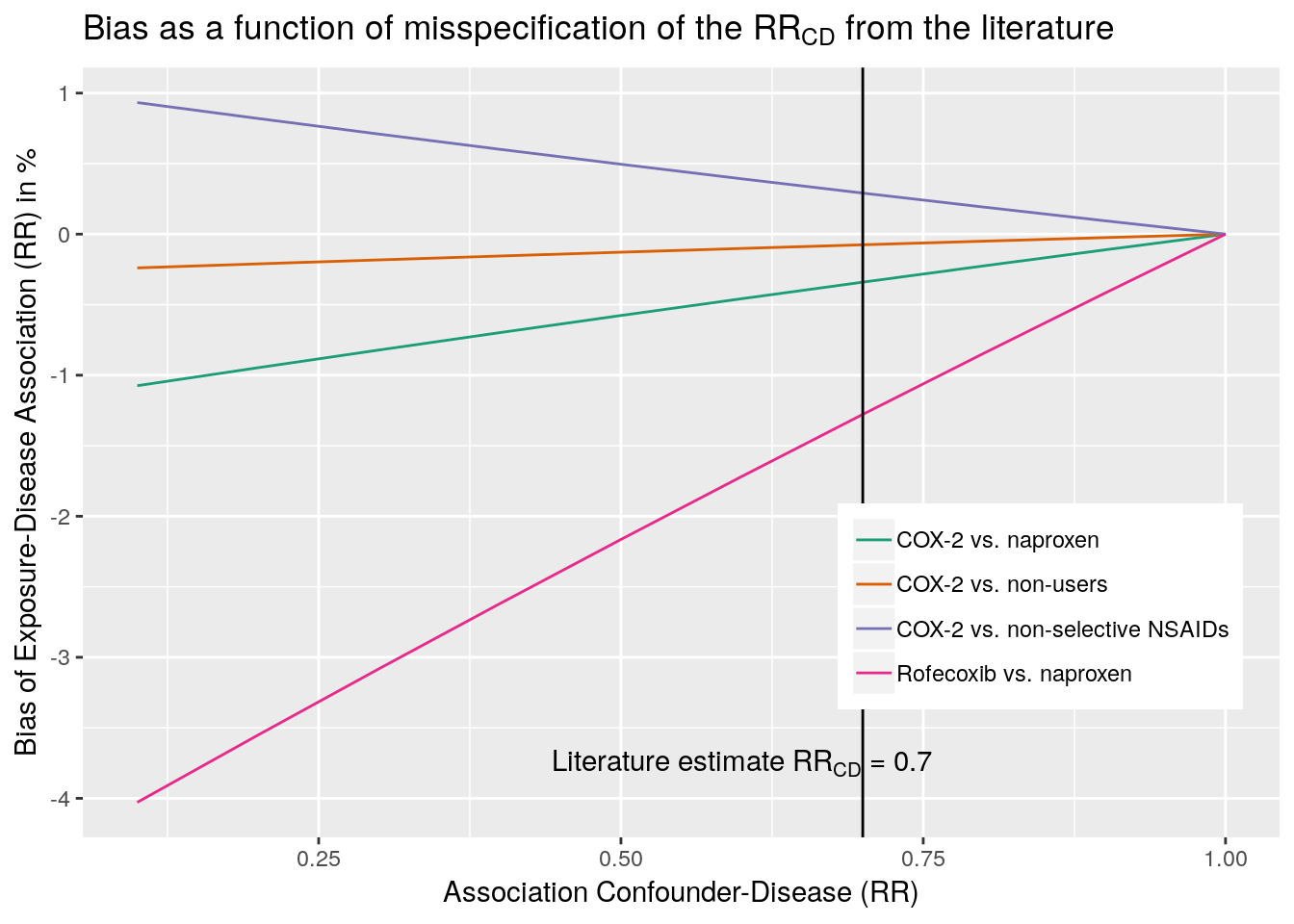# New in episensr 0.9.3 - Array approach for unmeasured confounders

New version of episensr available on CRAN! Besides now being easier to run multiple bias analysis, this new version offers the option to check the effect of residual confounding based on the array approach (Schneeweiss, 2006).

Even after controlling for confounding (in the design or analysis of a study), some residual confounding can still be present, because:

1. some confounding factors were not taken into account (not looked at, not adjusted for, or no data collected about them),
2. you only losely took into account that confounding (still presence of differences between groups after control by matching for example, or you lost precision on a confounding variable e.g. by categorizing a continuous variable),
3. subjects were not correctly classified to confounding variables.

## Simple sensitivity analyses in the absence of external information: Array approach

The array approach by Schneeweis provides a corrected RR based on the apparent, confounded, RR, the prevalence of the confounding factor among the exposed and unexposed, and the association between the confounder and the outcome.

Schneeweiss provides the (made-up) example of the association between TNF$$_\alpha$$ blocking agents and Non-Hodgkin (NH) Lymphoma in rheumatoid arthritis (RA) patients. Assuming an observed relative risk of 2, that 50% of RA patients have a more progressive immunologic disease, and that more progressive disease is more likely to lead to NH lymphoma:

library(episensr)
## Registered S3 methods overwritten by 'ggplot2':
##   method         from
##   [.quosures     rlang
##   c.quosures     rlang
##   print.quosures rlang
confounders.array(crude.risk = 2,
type = "binary",
bias_parms = c(2.5, 0.1, 0.5))
##
##
## Percent bias: -34.29
##
## Input Bias Parameters:
## ----------------------
##
##     RR(Confounder-Disease): 2.5
##   p(Confounder+|Exposure+): 0.1
##   p(Confounder+|Exposure-): 0.5

The function takes values of the observed risk (2.0); if the covariates are binary, continuous, or on a risk difference scale; the association between confounder and outcome (let’s say here 2.5); the prevalence of the confounder among the exposed (let’s say 0.1); and the prevalence of the confounder among the unexposed. The adjusted RR is 3.04.

However, it is more interesting if we could keep some factors constant in our analysis (the crude risk of 2.0, the prevalence among the unexposed, 0.5), and vary the imbalance of the hypothetical unoberved confounder (association confounder-outcome from 1 to 5.5; prevalence among exposed, from 0 to 1):

dat <- expand.grid(RR_CD = seq(1, 5.5, 0.5), P_C1 = seq(0, 1, 0.1))
dat$RR_adj <- apply(dat, 1, function(x) confounders.array(2, type = "binary", bias_parms = c(x, x, 0.5), print = FALSE)) Then adding fun with 3-d plots! library(lattice) library(grid) wireframe(RR_adj ~ RR_CD * P_C1, data = dat, xlab = "RR\nconfounder-outcome", ylab = "Prevalence confounder\namong exposed", zlab = "Adjusted\nRR", drape = TRUE, colorkey = TRUE, scales = list(arrows = FALSE, cex = .5, tick.number = 10, z = list(arrows = FALSE), distance = c(1.5, 1.5, 1.5)), zlim = 0:4, light.source = c(10, 0, 10), col.regions = rainbow(100, s = 1, v = 1, start = 0, end = max(1, 100 - 1) / 100, alpha = .8), screen = list(z = -60, x = -60), panel = function(...) { panel.wireframe(...) grid.text("Observed RR = 1\nPrevalence confounder\namong unexposed = 0.5", 0.125, 0.175, default.units = "native") })Good old lattice… But you can have even more fun with plotly: RR_CD <- seq(1, 5.5, 0.5) P_C1 <- seq(0, 1, 0.1) mat <- outer(RR_CD, P_C1, Vectorize(function (i, j) confounders.array(2, type = "binary", bias_parms = c(i, j, 0.5), print = FALSE))) library(plotly) plot_ly(x = ~P_C1, y = ~RR_CD, z = ~mat) %>% add_surface() %>% layout(scene = list(xaxis = list(title = "Pr conf. in exposed", nticks = 5), yaxis = list(title = "RR conf.-RA", nticks = 5), zaxis = list(title = "Adjusted RR", nticks = 5), aspectmode = "cube")) ## Using additional information: external adjustment You can also use additional information not available in the main study to adjust for the effect of confounding. Sometimes you can get information from a representative sample to quantify the imbalance risk factors that are measured among the exposed group; and from the literature (RCT, observational studies) for the association between the confounder and the outcome. We can use the example of Schneeweiss regarding the association between selective COX-2 inhibitor use and the incidence of MI. Say we assume an exposure-disease association of 1 (null hypothesis). The more the truth is away from the null, the more bias in our estimate. However the less relevant unmeasured confounders become. Let’s try: confounders.ext(RR = 1, bias_parms = c(0.1, 1.6, 0.1, 0.51)) ## ## Crude RR: 0.96 ## ## Percent bias: -4.03 ## ## Input Bias Parameters: ## ---------------------- ## ## RR(Confounder-Disease): 0.1 ## OR(Exposure category-Confounder): 1.6 ## p(Confounder): 0.1 ## p(Exposure): 0.51 Same here, the interest lies in using the function over a range of values, while keeping some other constant. And we can extend to several confounders: dat <- expand.grid(RR_CD = seq(0.1, 1, 0.1)) dat$nsaid <- apply(dat, 1,
function(x) confounders.ext(1,
bias_parms = c(x, 0.9, 0.1, 0.4),
print = FALSE))
dat$non_user <- apply(dat, 1, function(x) confounders.ext(1, bias_parms = c(x, 1.03, 0.09, 0.12), print = FALSE)) dat$naproxen <- apply(dat, 1,
function(x) confounders.ext(1,
bias_parms = c(x, 1.15, 0.09,
0.79),
print = FALSE))
dat\$rof_napro <- apply(dat, 1,
function(x) confounders.ext(1,
bias_parms = c(x, 1.6, 0.1, 0.51),
print = FALSE))
library(tidyverse)
## ── Attaching packages ────────────────────────────────── tidyverse 1.2.1 ──
## ✔ tibble  2.1.1     ✔ purrr   0.3.2
## ✔ tidyr   0.8.3     ✔ dplyr   0.8.1
## ✔ readr   1.3.1     ✔ stringr 1.4.0
## ✔ tibble  2.1.1     ✔ forcats 0.4.0
## ── Conflicts ───────────────────────────────────── tidyverse_conflicts() ──
## ✖ dplyr::filter() masks plotly::filter(), stats::filter()
## ✖ dplyr::lag()    masks stats::lag()
dat2 <- dat %>% gather(nsaid, non_user, naproxen, rof_napro,
key = "COX2", value = "bias_perc")
library(ggplot2)
ggplot(dat2, aes(x = RR_CD, y = bias_perc, group = COX2, colour = COX2)) +
geom_line() +
scale_colour_brewer(palette = "Dark2",
labels = c("COX-2 vs. naproxen", "COX-2 vs. non-users",
"COX-2 vs. non-selective NSAIDs",
"Rofecoxib vs. naproxen")) +
geom_vline(xintercept = 0.7) +
xlab("Association Confounder-Disease (RR)") +
ylab("Bias of Exposure-Disease Association (RR) in %") +
ggtitle(expression(paste("Bias as a function of misspecification of the ", RR[CD], " from the literature"))) +
theme(legend.position = c(.8, .3),
legend.title = element_blank()) +
annotate("text", label = expression(paste("Literature estimate ", RR[CD], " = 0.7")), x = 0.6, y = -3.75)
## Warning in is.na(x): is.na() applied to non-(list or vector) of type
## 'expression'